Test 2 Section 3 #7

This is one of my favorite questions because there are a couple fun paths to take: one with pure exponent rules, and one with exponent rules and difference of two squares. So fun! (I understand if you don’t share my enthusiasm.)

First, the exponent-rules-only method. We need to take what we’re given and simplify it enough that we can stop worrying about x and focus exclusively on a and b. To do that, we first need to remember that when we raise a power to a power, we multiply the powers. In other words: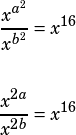From there, we need to remember that when we divide exponential expressions with the same base, we subtract the exponents.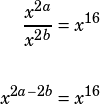From there, we’re good to eliminate x and just say that. Since the question asks us for the value of, just divide that equation by 2 to get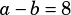.

Going the second way involves the division exponent rule but not the power-to-a-power rule. Skip right to the division rule:From there, we can say that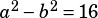and use our difference of two squares skills.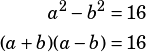The question tells us that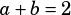, so let’s substitute: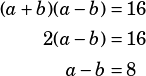So there you go—two fun ways to get this one done. Which do you prefer?